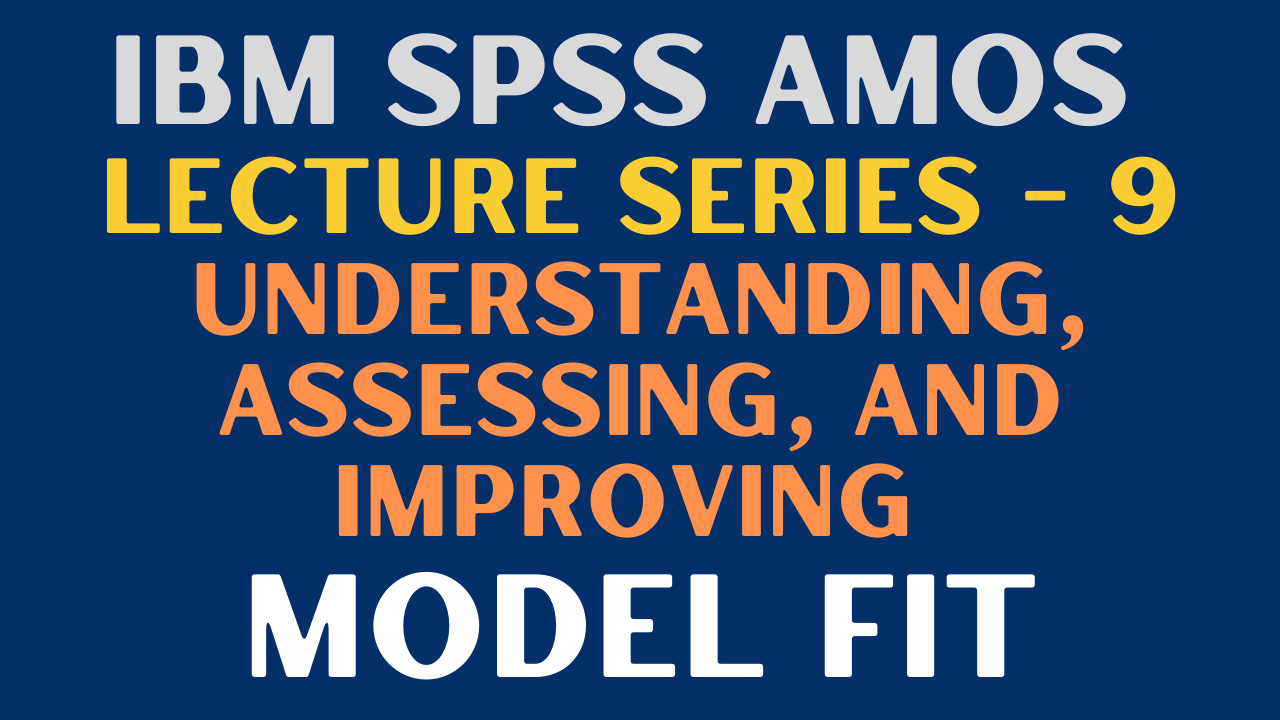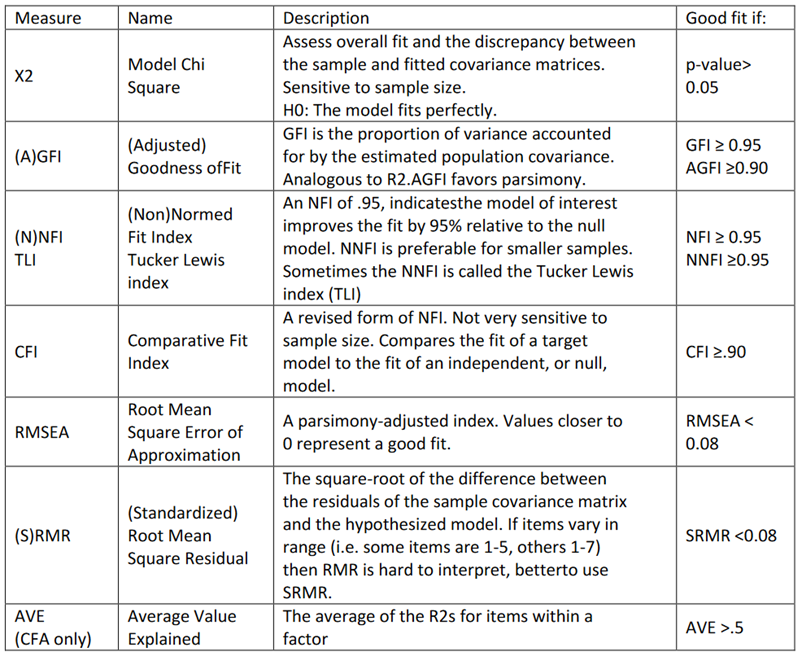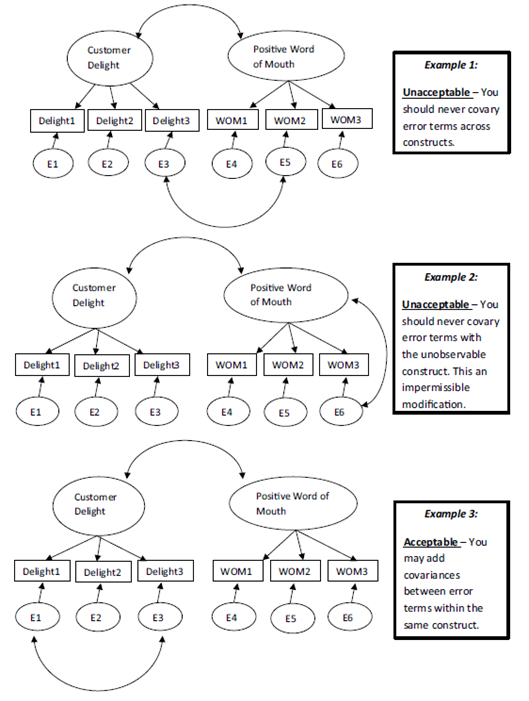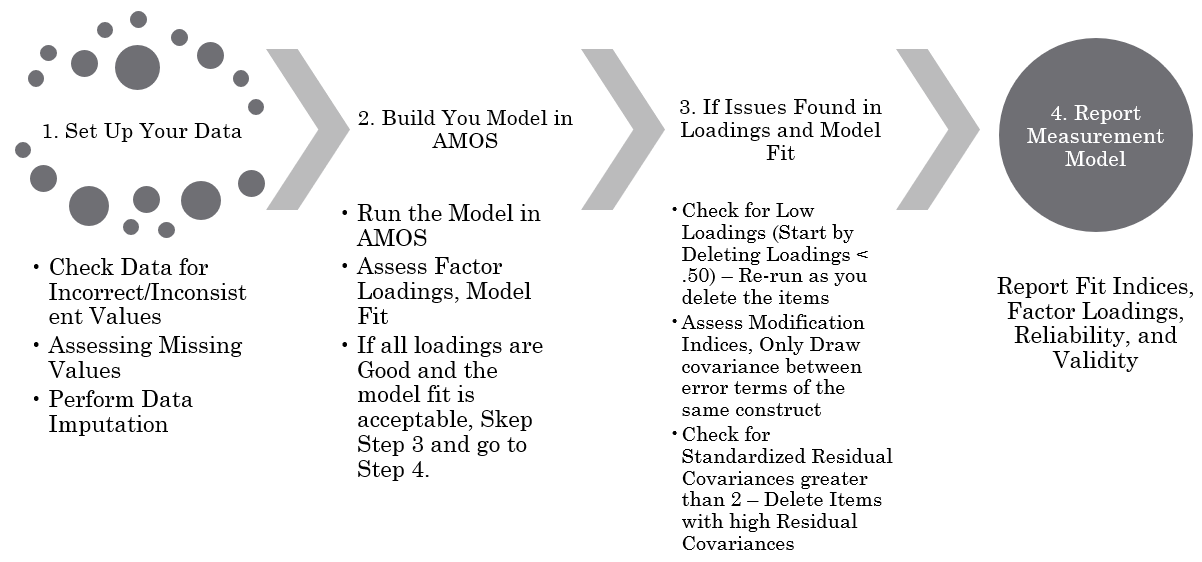# Understanding, Assessing, and Improving SPSS AMOS Model Fit### IBM SPSS AMOS Series - 9

SPSS AMOS Lecture Series: Understanding, Assessing, and Improving Model Fit in AMOS. The focus of the session is on helping research scholars understand how to fit a model in AMOS.

## Understanding Model Fit

• The researcher first specifies a model based on theory, then determines how to measure constructs, collects data, and then inputs the data into the SEM software package.
• The package fits the data to the specified model and produces the results, which include overall model fit statistics and parameter estimates.
• Model fit is the feasibility of the model with the data.

## Model Fit CriteriaSource: Principles and Practice of Structural Equation Modeling. Rex B. Kline. 2005

## Improving Model Fit - Modification Indices

• Check for modification indices. However, be cautious, not every suggested covariance shall be drawn.
• Assess Standardized Residual Covariance (Estimates Section)Source: Applied structural equation modeling using AMOS: Basic to advanced techniques by Collier, J. E. (2020).

• In instances where an unobserved variable has multiple indicators, you might want to correlate the error terms if it is theoretically justifiable and helps to explain the variance within the construct.
• Frequently, indicators within a construct are very similar to one another and there is redundancy between the indicators. How big does the modification indices value need to be to add another covariance?
• Consider any valid modification suggestion if the chi-square value is not changing by at least 10. A chi-square value of 10 at the expense of one degree of freedom is almost significant at a .001 level.
• I look for instances where the change is extreme. It is not uncommon to have a modification suggestion greater than 20 in large models.
• An equal concern for multiple high modification indices within a construct is the possibility that this shared unexplained error is the result of a method bias in how the data was collected.

## Improving Model Fit - Standardized Residual Covariances

• Standardized residual covariances indicate the standardized differences between the proposed covariances based on the model and the observed covariance matrix computed based on the collected data.
• Significant values of standardized covariance indicate significant differences in covariance between proposed model based computed covariance and observed covariance and affects the overall goodness of fit.
• Although, the standardized residual covariance are like modification indices, they can directly be addressed through deletion of the concerned item. Therefore, the significant standardized residuals may be treated only after taking care of modification indices.
• Look for standardized residual covariance estimates greater than 2 to identify particular covariances which seem associated with significant discrepancies of the given model from a perfect model.

## BE Cautious!

• Do not Delete an item which is central to your construct and can affect Content Validity
• Make sure you have at least 3 items per Construct.

## Process of Assessing Model Fit## References

• Collier, J. E. (2020). Applied structural equation modeling using AMOS: Basic to advanced techniques. Routledge.
• Awang, Z. (2015). A Handbook on SEM (2nd Edition). Malaysia.